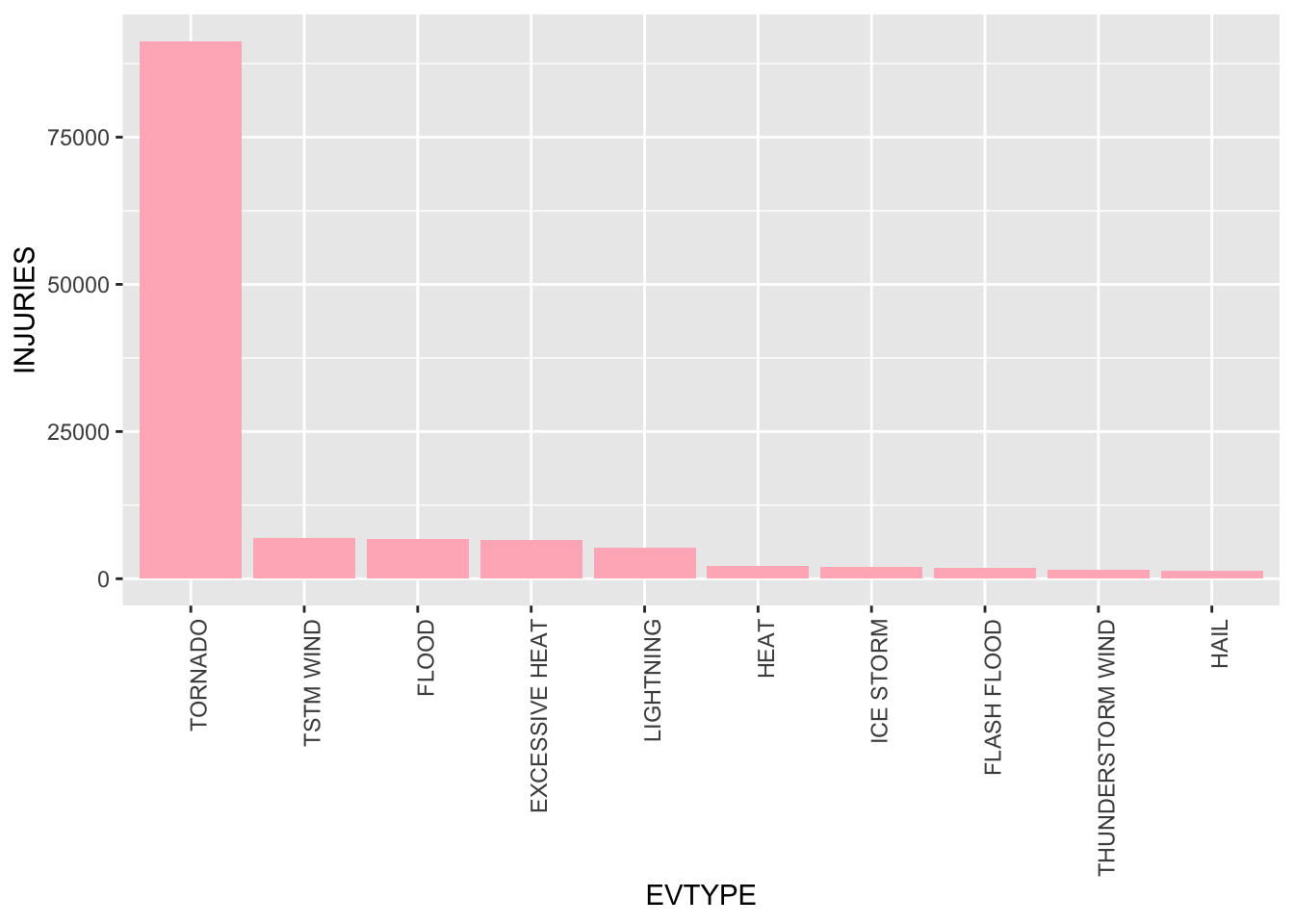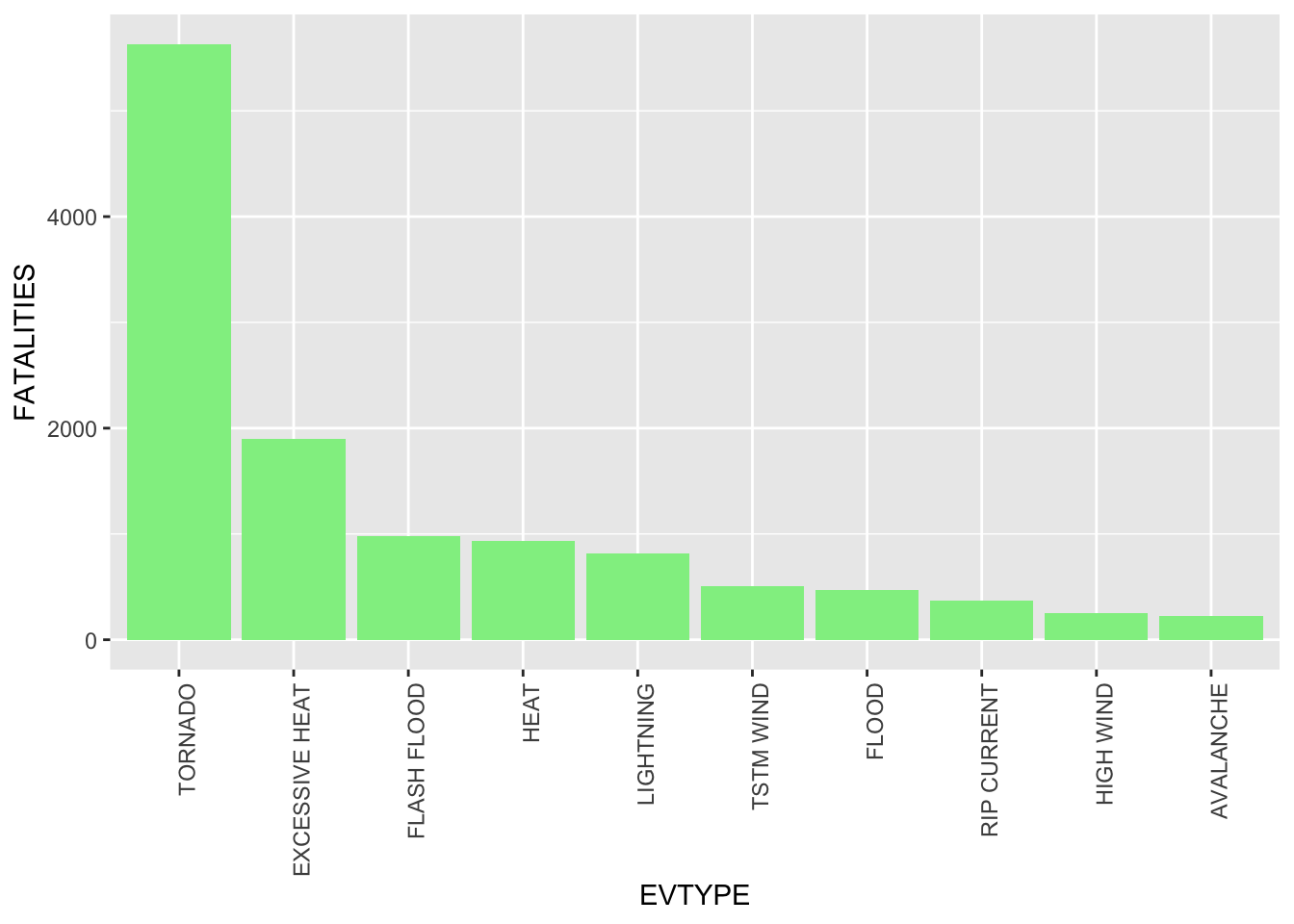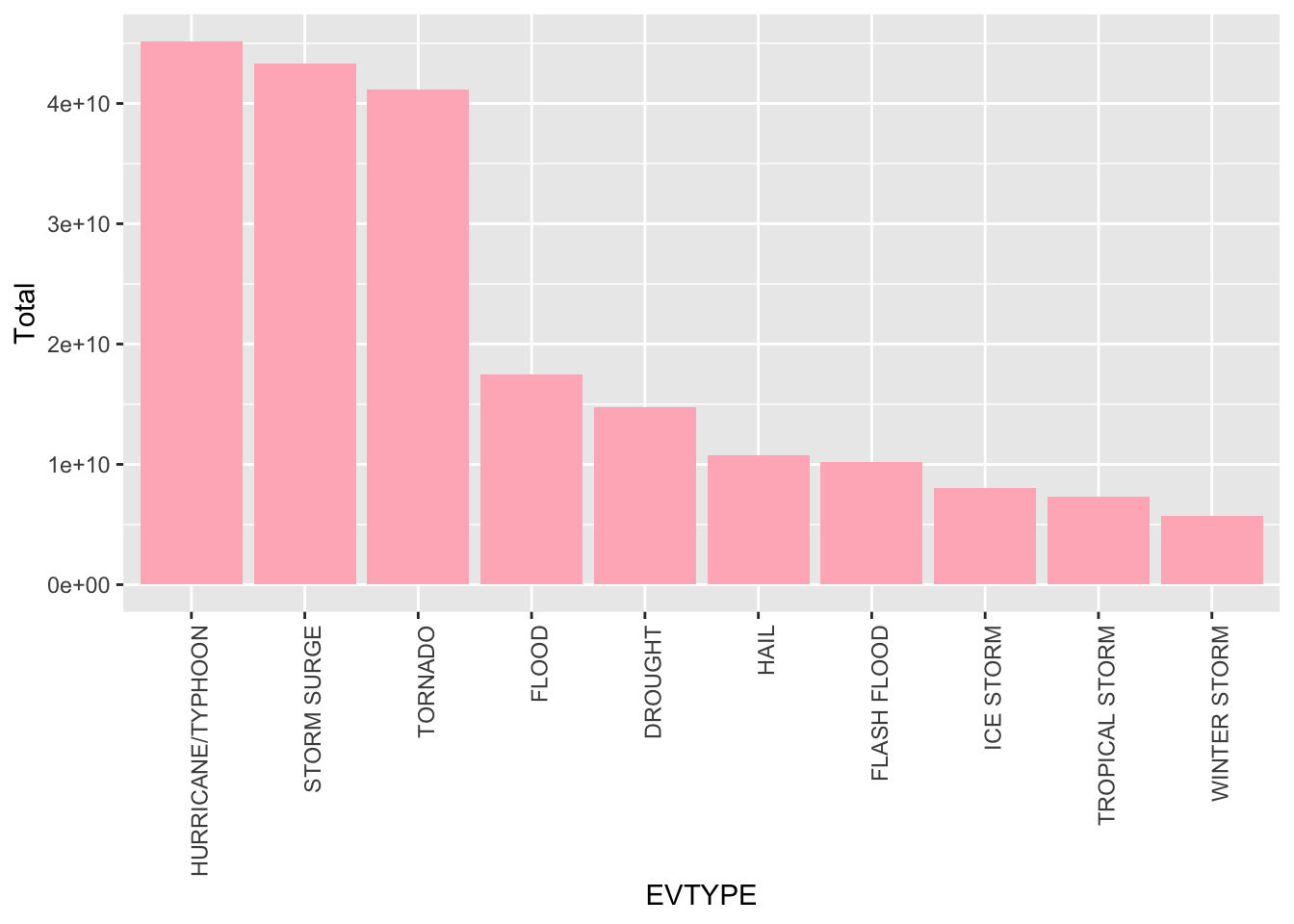#Synopsis In this project, we investigate the effect of many different types weather events on resulting population health (through injuries and fatalities) and on the economy (through property damage and crop damage). We found that tornados are the most harmful weather event in terms of both injuries and fatalities. Hurricanes had the greatest economic consequences in terms of property damage and crop damage. The method of how this information was acquired is shown below along with some helpful figures.

We first load all useful libraries, the data, and subset based on population health and economic consequences.

library(dplyr)
##
## Attaching package: 'dplyr'
## The following objects are masked from 'package:stats':
##
##     filter, lag
## The following objects are masked from 'package:base':
##
##     intersect, setdiff, setequal, union
library(ggplot2)
tidySD <- stormdata[,c('EVTYPE','FATALITIES','INJURIES', 'PROPDMG', 'PROPDMGEXP', 'CROPDMG', 'CROPDMGEXP')] #population damage and economic consequences

# Population Health

## Aggregating and Ordering Data

x <- as.data.frame(aggregate(INJURIES ~ EVTYPE,tidySD,sum))
y <- as.data.frame(aggregate(FATALITIES ~ EVTYPE, tidySD, sum))

x <- x %>% arrange(desc(x$INJURIES)) %>% mutate(EVTYPE=factor(EVTYPE, levels=EVTYPE)) y <- y %>% arrange(desc(y$FATALITIES)) %>% mutate(EVTYPE=factor(EVTYPE, levels=EVTYPE))

## Plots

Here we plot both top ten event types resulting in the highest injuries and fatalities.

#top ten observations for plotting
ggplot(x[1:10,],aes(x=EVTYPE,y=INJURIES)) + geom_bar(stat="identity", fill= " light pink") + theme(axis.text.x = element_text(angle = 90, hjust=1))ggplot(y[1:10,],aes(x=EVTYPE,y=FATALITIES)) + geom_bar(stat="identity", fill= " light green") + theme(axis.text.x = element_text(angle = 90, hjust=1))# Economic Consequences

## Processing Data

Processing data such that the property damage and crop damage value have the exponents accounted for.

tidySD[tidySD$PROPDMGEXP == "H", ]$PROPDMG <- tidySD[tidySD$PROPDMGEXP == "H", ]$PROPDMG * 100
tidySD[tidySD$PROPDMGEXP == "K", ]$PROPDMG <- tidySD[tidySD$PROPDMGEXP == "K", ]$PROPDMG * 1000
tidySD[tidySD$PROPDMGEXP == "M", ]$PROPDMG <- tidySD[tidySD$PROPDMGEXP == "M", ]$PROPDMG * 10^6
tidySD[tidySD$PROPDMGEXP == "B", ]$PROPDMG <- tidySD[tidySD$PROPDMGEXP == "B", ]$PROPDMG * 10^9

tidySD[tidySD$CROPDMGEXP == "H", ]$PROPDMG <- tidySD[tidySD$CROPDMGEXP == "H", ]$CROPDMG * 100
tidySD[tidySD$CROPDMGEXP == "K", ]$PROPDMG <- tidySD[tidySD$CROPDMGEXP == "K", ]$CROPDMG * 10^3
tidySD[tidySD$CROPDMGEXP == "M", ]$PROPDMG <- tidySD[tidySD$CROPDMGEXP == "M", ]$CROPDMG * 10^6
tidySD[tidySD$CROPDMGEXP == "B", ]$PROPDMG <- tidySD[tidySD$CROPDMGEXP == "B", ]$CROPDMG * 10^9

## Aggregating and Ordering Data

a <- as.data.frame(aggregate(PROPDMG ~ EVTYPE,tidySD,sum))
b <- as.data.frame(aggregate(CROPDMG ~ EVTYPE, tidySD, sum))

totaldamages <- merge(a,b, by = intersect(names(a),names(b)))
totaldamages <- totaldamages %>% mutate(Total = PROPDMG + CROPDMG) %>% arrange(desc(Total)) %>% mutate(EVTYPE=factor(EVTYPE, levels=EVTYPE))

Plotting total damages based on event type.

ggplot(totaldamages[1:10,],aes(x=EVTYPE,y=Total)) + geom_bar(stat="identity", fill= " light pink") + theme(axis.text.x = element_text(angle = 90, hjust=1))# Results

Quick view on top five events for public health and economic effects.

head(x)
##           EVTYPE INJURIES
## 2      TSTM WIND     6957
## 3          FLOOD     6789
## 4 EXCESSIVE HEAT     6525
## 5      LIGHTNING     5230
## 6           HEAT     2100
head(y)
##           EVTYPE FATALITIES
## 6      TSTM WIND        504
head(totaldamages)
##              EVTYPE     PROPDMG   CROPDMG       Total
## 6              HAIL 10761317275 579596.28 10761896871